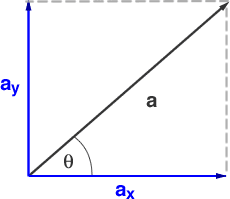# Calculating electric field

fymdmk

## Homework Statement

In the figure (attached), find the magnitude and direction of the electric field at points A, B, C and D.

E = kQ / r^2

## The Attempt at a Solution

I'm just a little lost with the directions/if I need to be including angles
so for point A:
E = k 7.5e^5C / 0.25m + k 7.5e^5C / ?
is it 0.55m (as figured out by Pythagorean theorem) or would it be the X is 0.55cos26 and Y is 0.55 sin26 + the other charge (since it is only in the Y direction)

thanks!!

#### Attachments

•physics diagram.PNG
3 KB · Views: 376

## Answers and Replies

Homework Helper
E = k 7.5e^5C / 0.25m + k 7.5e^5C / ?
Electric field is a vector quantity. You don't in general add two electric fields like you did above. Do you know how to add two vectors?

fymdmk
right - okay so then its probably my second guess? splitting it into X and Y?

so point A would have the Y of the first 75 charge plus the Y of the second 75 charge which would be sin26 0.55 and then the X's would just be the cos26 0.55 of the second 75 charge? does that make sense

Homework Helper
so point A would have the Y of the first 75 charge plus the Y of the second 75 charge which would be sin26 0.55 and then the X's would just be the cos26 0.55 of the second 75 charge? does that make sense
You don't elaborate your second attempt in a good mathematical language. You merely guessed that some expression of the form 0.55 cos 26 is involved, which I don't know how you will make it relevant with the problem.
To start, let's first consider the field at point A. Then write out the field vectors from each charge at this point.

fymdmk
sorry! okay so point A:

charge 1 (bottom left) would be: k (7.5e^5C)/0.25m in the upwards direction

charge 2 (bottom right) would be split into 2 vectors.
1) find the hypotenuse of the triangle that would get from the charge to point A. this value would be x = sqr rt (50^2 + 25^2) = 55cm = 0.55m
2) find the angle between the 50cm bottom and 55cm hypotanuse. If I use cos that would be cos^-1 (50/55) = 25 degrees.
3) split the bottom charge into 2 vectors:
vertical vector = k (7.5e^5 C) / 0.55 sin25 in the upwards direction
horizontal vector = k (7.5e^5 C) / 0.55 cos25 in the leftwards direction

so in order to get the total I would add the two vertical components of the different charges and then...I get stuck, do I need to make another triangle?

Homework Helper
k (7.5e^5C)/0.25
The magnitude of electric field from a point charge is inversely proportional to the square of the distance between the source point and the observation point.
k (7.5e^5 C) / 0.55 sin25
Where is the ##\sin 25^o## located, in the denominator or numerator? Also, take my first statement above into account.

fymdmk
sorry I forgot that the direction is squared, so it would be 0.25 (or 055) ^2

is the sin25 in the numerator then? because it is affecting the charge?

that still leaves me with 2 upwards vertical components, and 1 left horizontal component - so then do I make a new triangle to get the total and direction of the electric field on A?

Homework Helper
is the sin25 in the numerator then?
I was asking you. Ok, let's say we have a vector ##\mathbf{a} = a_x\hat{i} + a_y\hat{j}## (see picture below)the magnitude of ##\mathbf{a}## is ##a = \sqrt{a_x^2+a_y^2}##. Given this information, how does ##a_x## and ##a_y## look like in terms of ##a## and ##\theta##?

fymdmk
okay so sin25 is definitely in the numerator, I see what you're saying.

and I think I've got that ax would be a acostheta and ay would be asintheta. so I add my two vertical vectors, and create a triangle using my combined two vertical vectors tip to toe with the horizontal vector, and find the hypotenuse which would be the total electric field magnitude, and then solve the new angle theta which would be the direction

Homework Helper
I think I've got that ax would be a acostheta and ay would be asintheta.
Yes.
so I add my two vertical vectors, and create a triangle using my combined two vertical vectors tip to toe with the horizontal vector, and find the hypotenuse which would be the total electric field magnitude, and then solve the new angle theta which would be the direction
Yes.

•gracy
fymdmk
awesome, thanks!